# Plot types#

Overview of many common plotting commands in Matplotlib.

Note that we have stripped all labels, but they are present by default. See the gallery for many more examples and the tutorials page for longer examples.

## Basic#

Basic plot types, usually y versus x.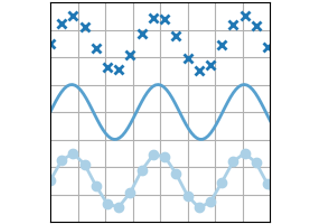plot(x, y)

plot(x, y)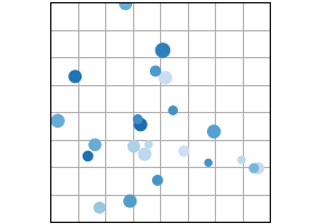scatter(x, y)

scatter(x, y)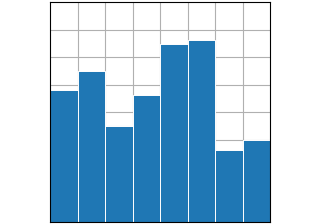bar(x, height)

bar(x, height)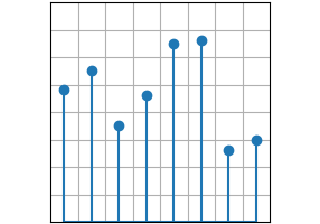stem(x, y)

stem(x, y)step(x, y)

step(x, y)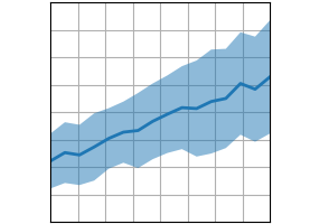fill_between(x, y1, y2)

fill_between(x, y1, y2)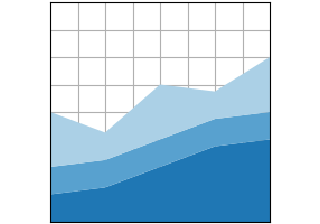stackplot(x, y)

stackplot(x, y)

## Plots of arrays and fields#

Plotting for arrays of data `Z(x, y)` and fields `U(x, y), V(x, y)`.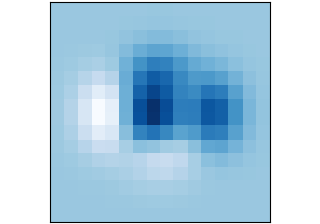imshow(Z)

imshow(Z)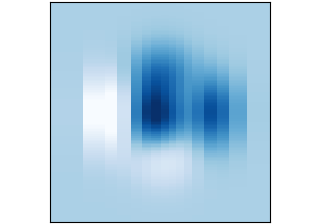pcolormesh(X, Y, Z)

pcolormesh(X, Y, Z)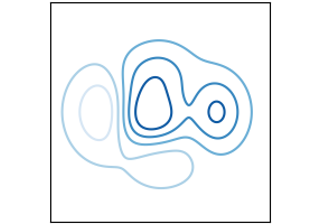contour(X, Y, Z)

contour(X, Y, Z)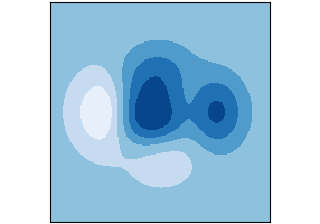contourf(X, Y, Z)

contourf(X, Y, Z)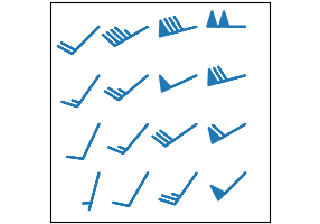barbs(X, Y, U, V)

barbs(X, Y, U, V)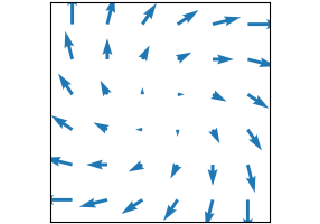quiver(X, Y, U, V)

quiver(X, Y, U, V)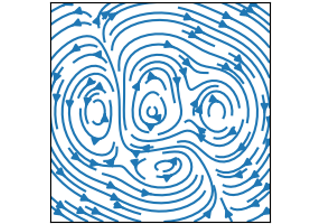streamplot(X, Y, U, V)

streamplot(X, Y, U, V)

## Statistics plots#

Plots for statistical analysis.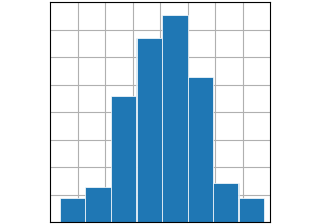hist(x)

hist(x)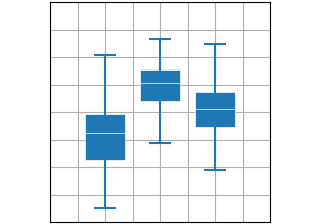boxplot(X)

boxplot(X)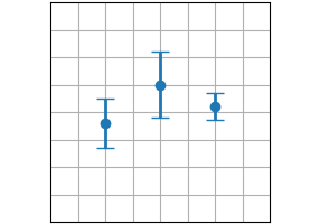errorbar(x, y, yerr, xerr)

errorbar(x, y, yerr, xerr)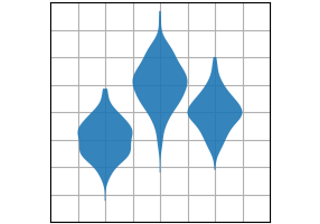violinplot(D)

violinplot(D)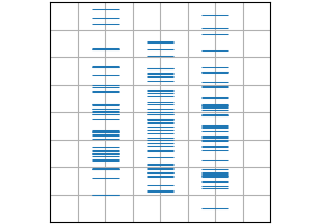eventplot(D)

eventplot(D)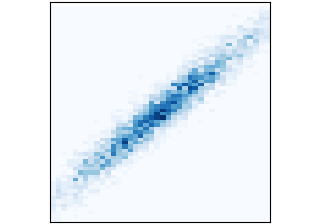hist2d(x, y)

hist2d(x, y)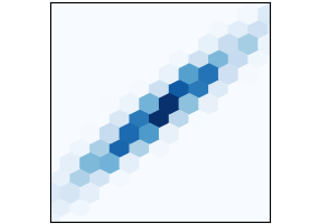hexbin(x, y, C)

hexbin(x, y, C)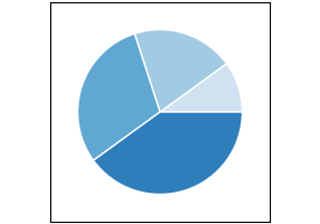pie(x)

pie(x)

## Unstructured coordinates#

Sometimes we collect data `z` at coordinates `(x,y)` and want to visualize as a contour. Instead of gridding the data and then using `contour`, we can use a triangulation algorithm and fill the triangles.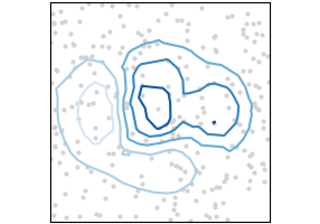tricontour(x, y, z)

tricontour(x, y, z)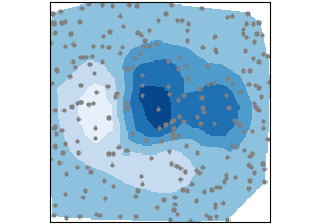tricontourf(x, y, z)

tricontourf(x, y, z)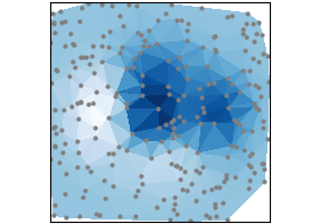tripcolor(x, y, z)

tripcolor(x, y, z)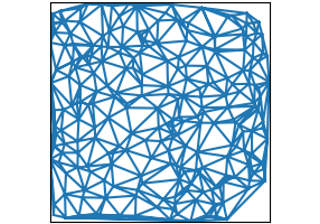triplot(x, y)

triplot(x, y)

Gallery generated by Sphinx-Gallery